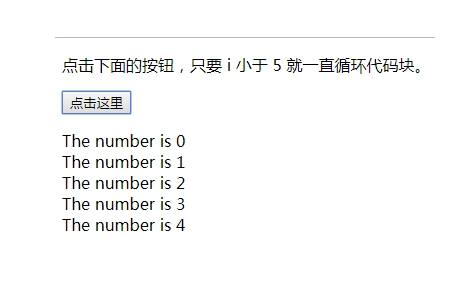JavaScript While 循环

while 循环

While 循环会在指定条件为真时循环执行代码块。

```while (条件)  {
需要执行的代码
}```

## 实例

```<script>
function myFunction()
{
var x="",i=0;
while (i<5)
{
x=x + "The number is " + i + "<br>";
i++;
}
document.getElementById("demo").innerHTML=x;
}
</script>```do/while 循环

do/while 循环是 while 循环的变体。该循环会执行一次代码块，在检查条件是否为真之前，然后如果条件为真的话，就会重复这个循环。

```do
{
需要执行的代码
}
while (条件);```

## 实例

```<script>
function myFunction()
{
var x="",i=0;
do
{
x=x + "The number is " + i + "<br>";
i++;
}
while (i<5)
document.getElementById("demo").innerHTML=x;
}
</script>```for 语句实例

## 实例

```<script>
cars=["BMW","Volvo","Saab","Ford"];
var i=0;
for (;cars[i];)
{
document.write(cars[i] + "<br>");
i++;
}
</script>```

while 语句实例

## 实例

```<script>
cars=["BMW","Volvo","Saab","Ford"];
var i=0;
while (cars[i])
{
document.write(cars[i] + "<br>");
i++;
}
</script>```